# 深度学习的数学（三）：神经元工作的数学表示## 简化神经元的图形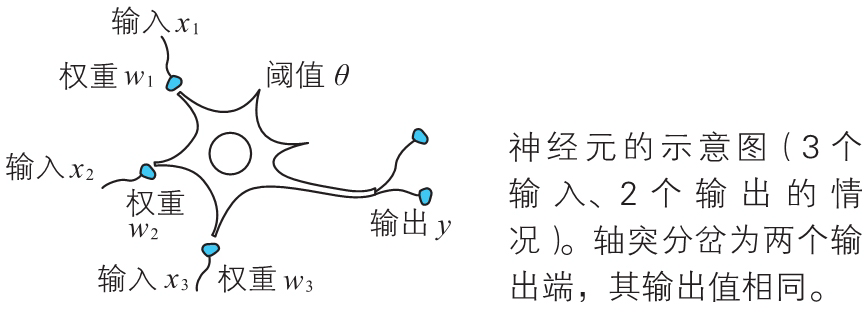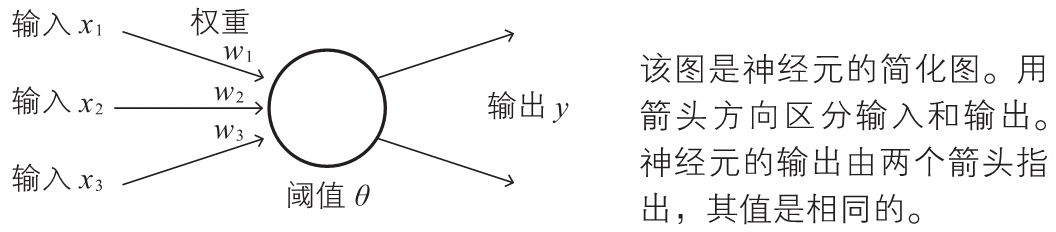## 激活函数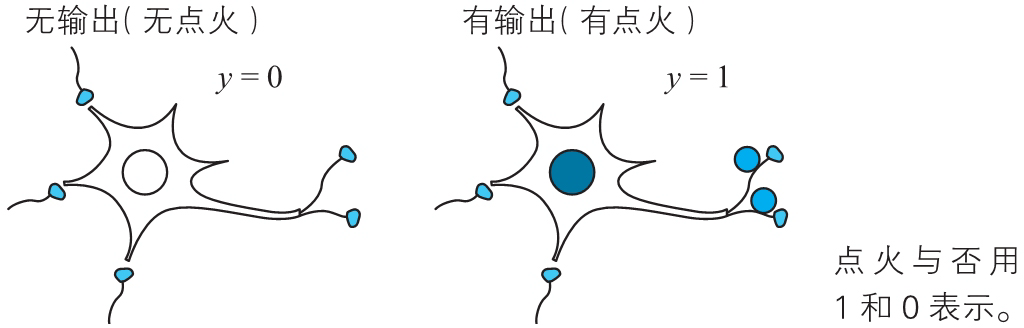$y=a(w_1x_1+w_2x_2+w_3x_3-\theta)\quad\quad\quad\quad\quad\quad\quad\quad(2)$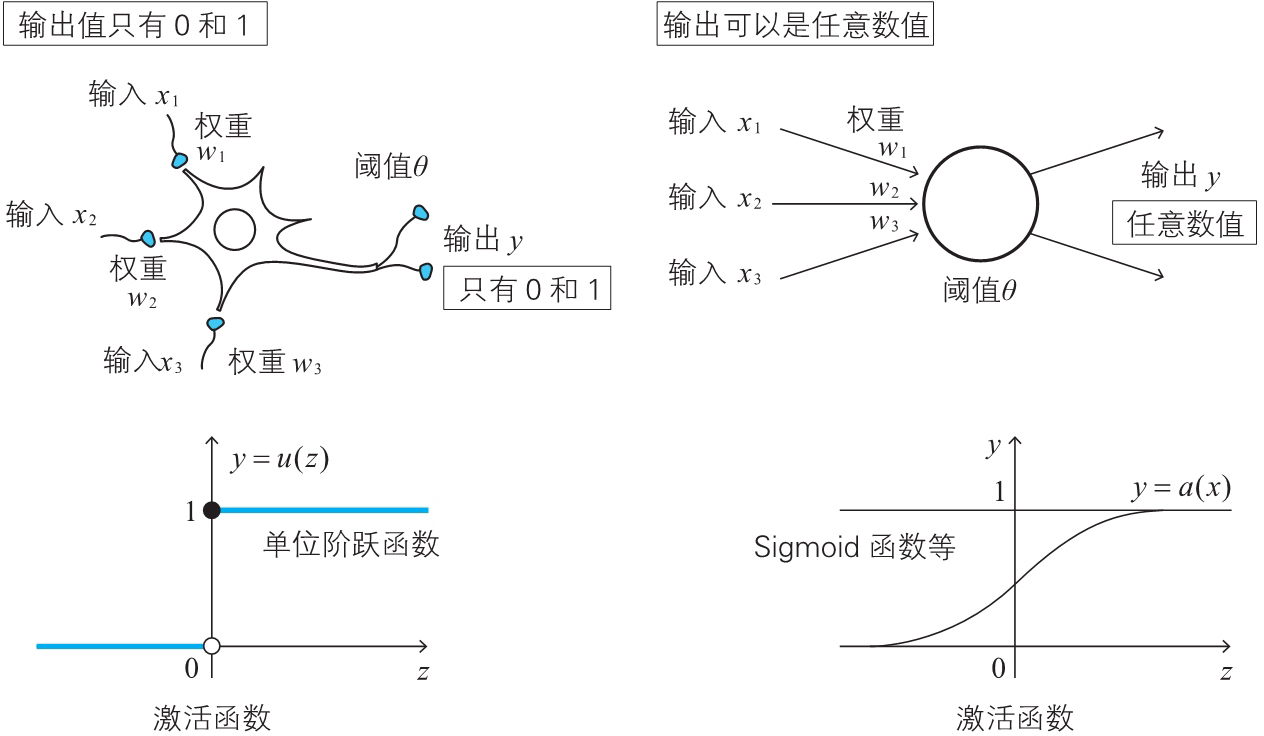## Sigmoid 函数

$\sigma(z)=\frac{1}{1+{\rm e}^{-z}}~~({\rm e}=2.718281\dots)\quad\quad\quad\quad\quad\quad\quad\quad(3)$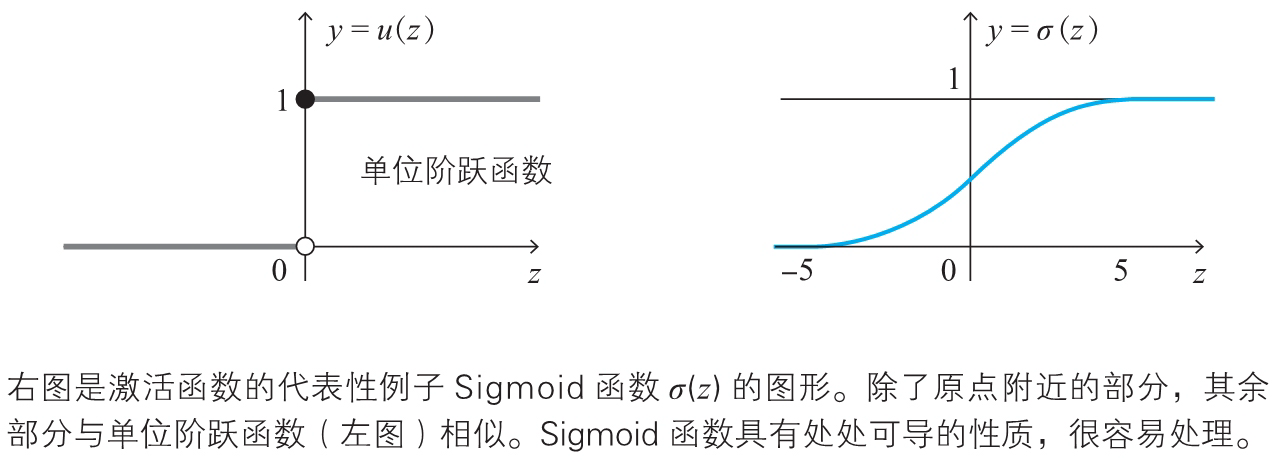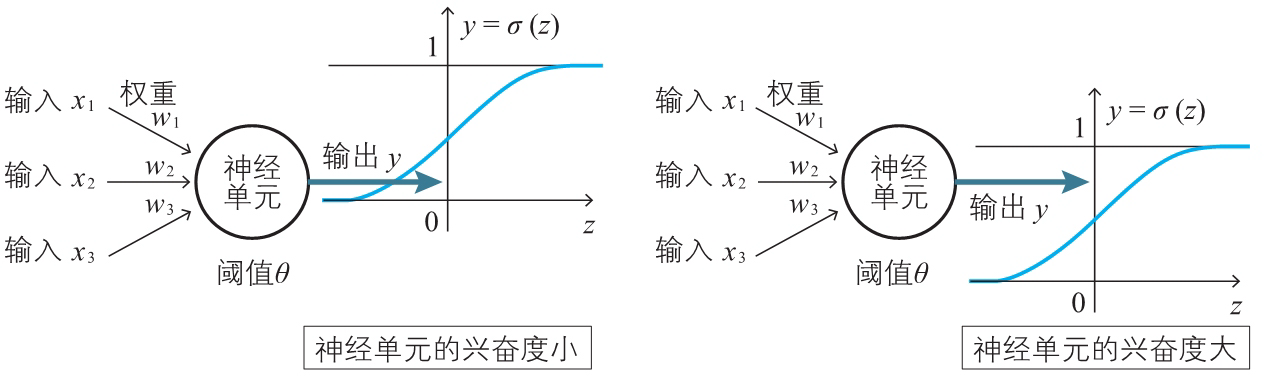## 偏置

$y=a(w_1x_1+w_2x_2+w_3x_3-\theta)\quad\quad\quad\quad\quad\quad\quad\quad(2)$

$y=a(w_1x_1+w_2x_2+w_3x_3+b)\quad\quad\quad\quad\quad\quad\quad\quad(4)$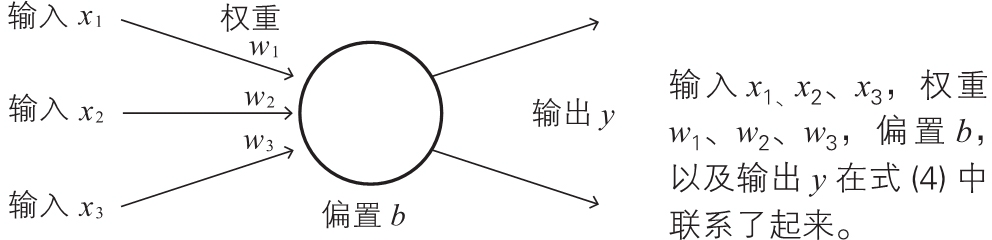$z=w_1x_1+w_2x_2+w_3x_3+b\quad\quad\quad\quad\quad\quad\quad\quad(5)$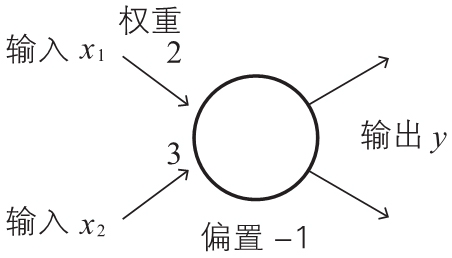0.2 0.1
0.6 0.5

0.2 0.1 2×0.2 + 3×0.1 - 1 = -0.3 0.43
0.6 0.5 2×0.6 + 3×0.5 - 1 = 1.7 0.84

$z=w_1x_x+w_2x_2+w_3x_3+b\times1\quad\quad\quad\quad\quad\quad\quad\quad(6)$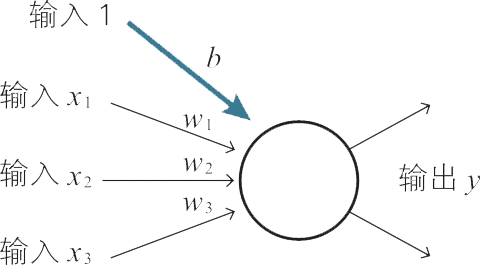$(w_1,w_2,w_3,b)(x_1,x_2,x_3,1)$